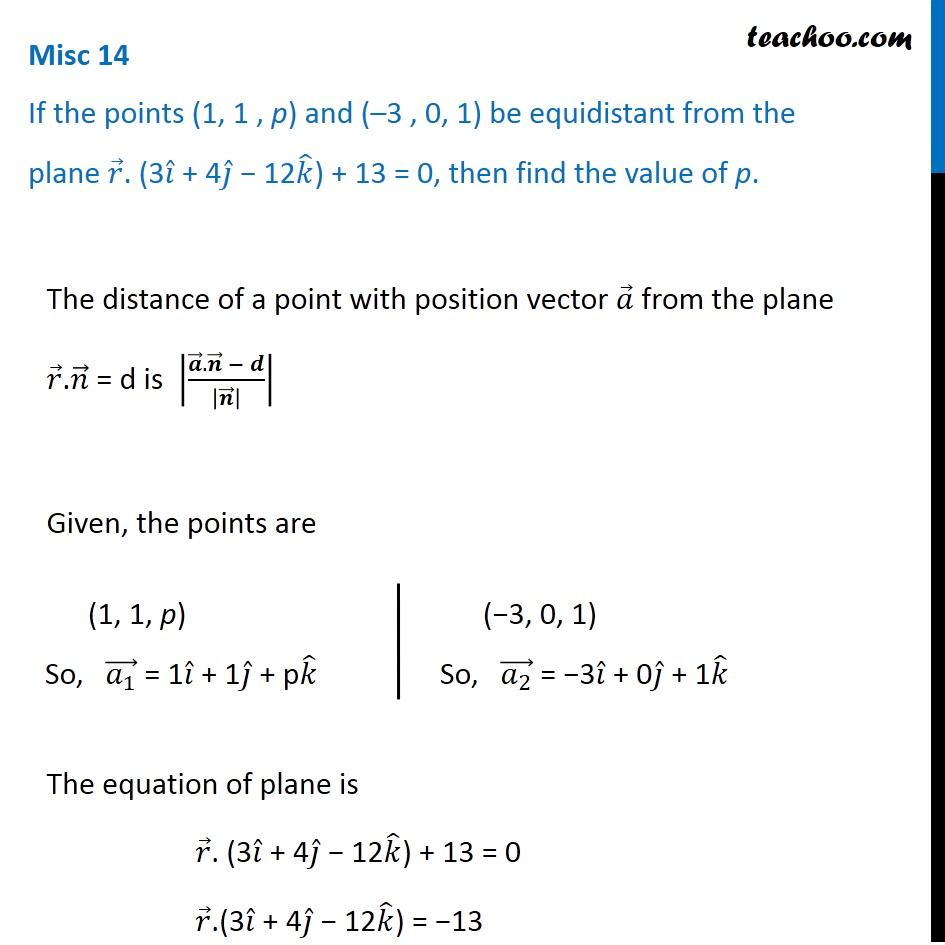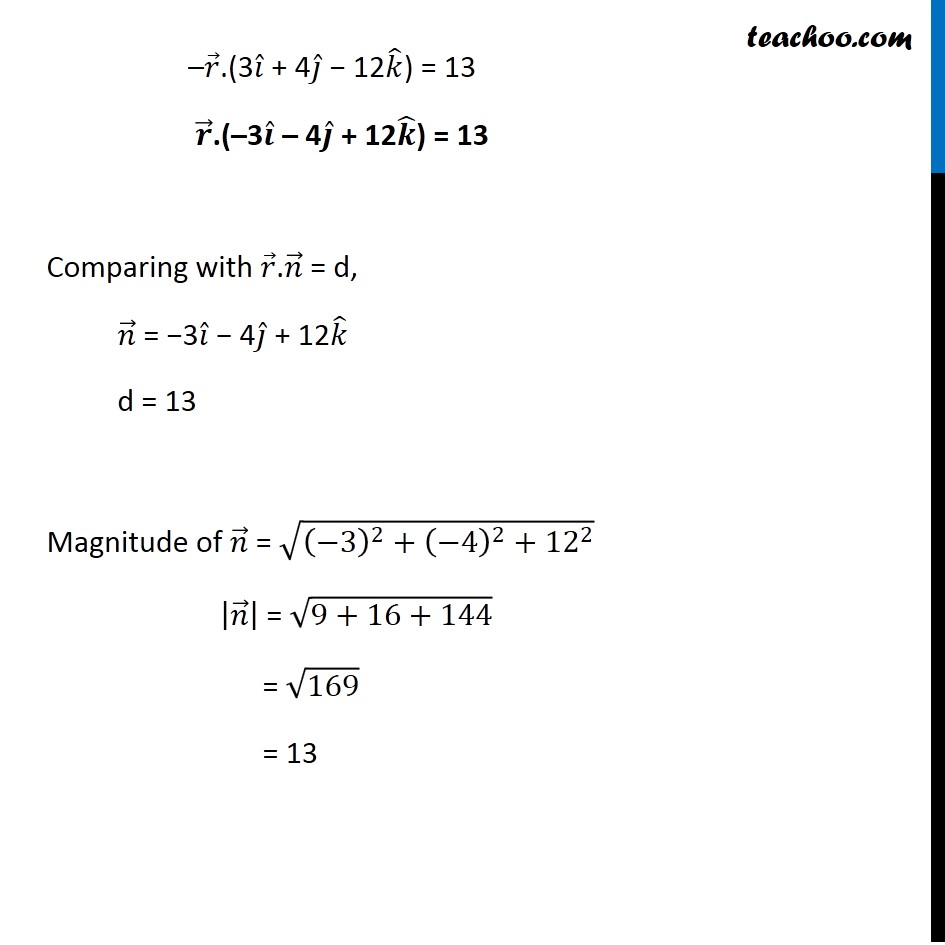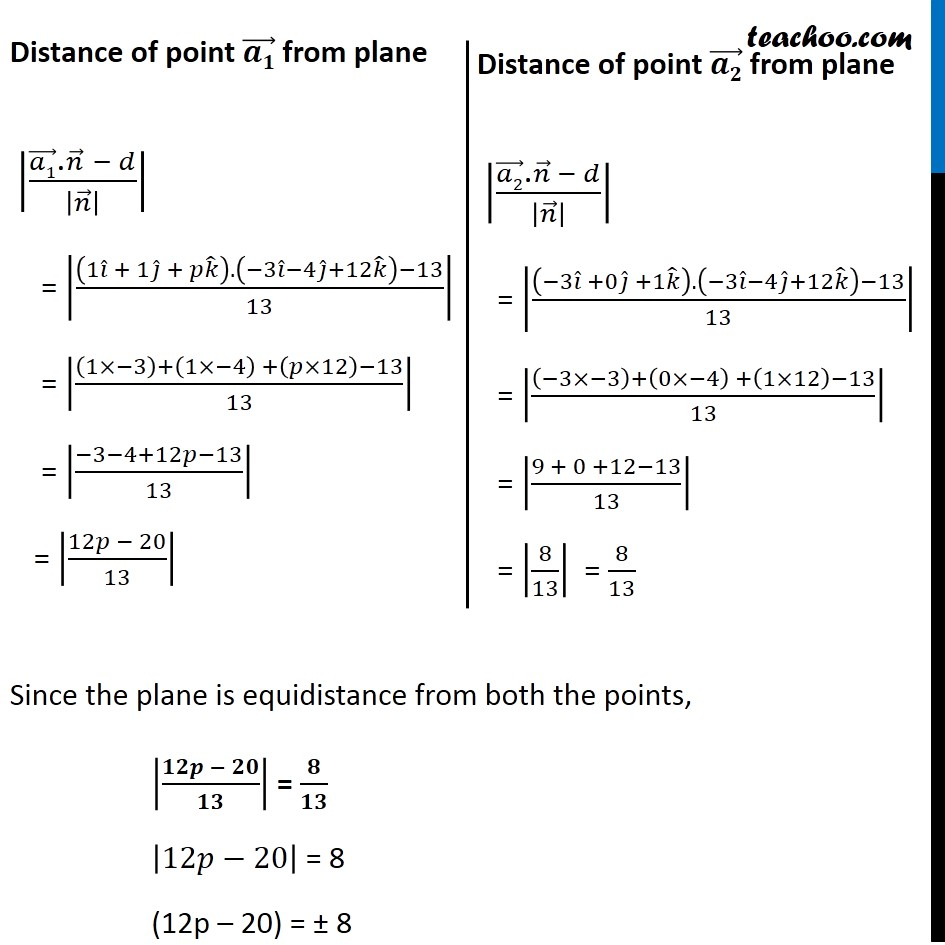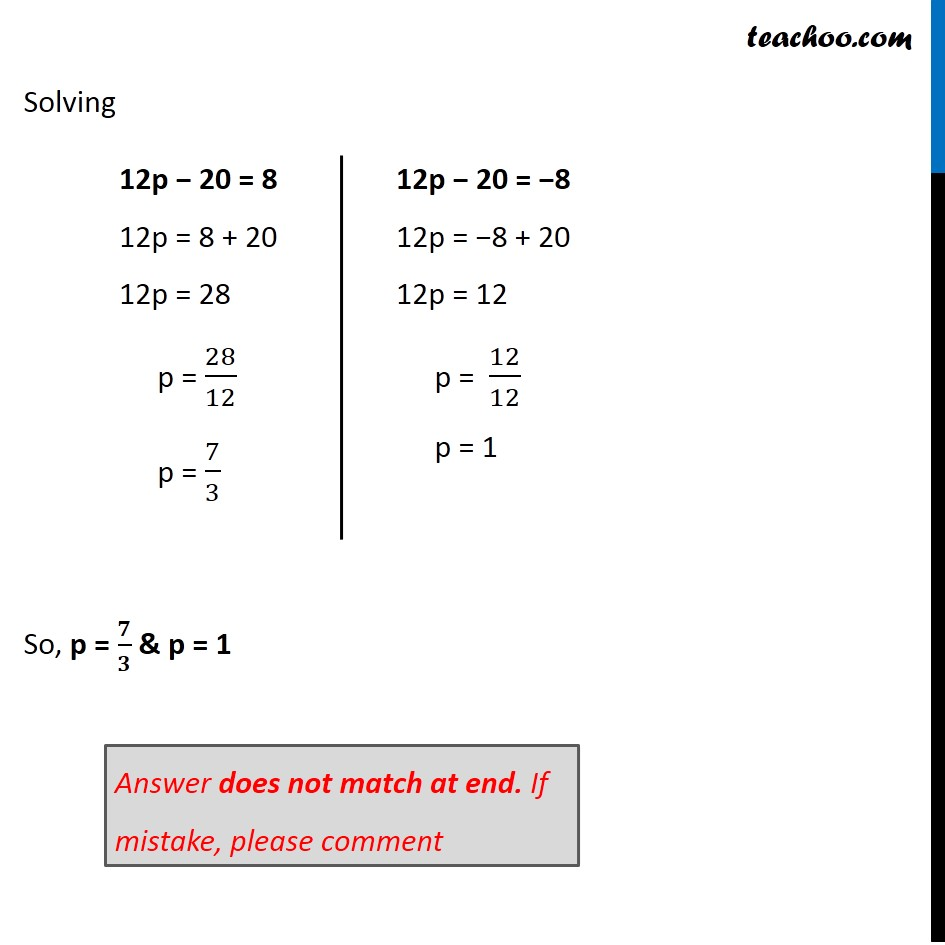Miscellaneous

Chapter 11 Class 12 Three Dimensional Geometry
Serial order wiseLearn in your speed, with individual attention - Teachoo Maths 1-on-1 Class

### Transcript

Question 10 If the points (1, 1 , p) and (–3 , 0, 1) be equidistant from the plane 𝑟 ⃗. (3𝑖 ̂ + 4𝑗 ̂ − 12𝑘 ̂) + 13 = 0, then find the value of p. The distance of a point with position vector 𝑎 ⃗ from the plane 𝑟 ⃗.𝑛 ⃗ = d is |(𝒂 ⃗.𝒏 ⃗ − 𝒅)/|𝒏 ⃗ | | Given, the points are The equation of plane is 𝑟 ⃗. (3𝑖 ̂ + 4𝑗 ̂ − 12𝑘 ̂) + 13 = 0 𝑟 ⃗.(3𝑖 ̂ + 4𝑗 ̂ − 12𝑘 ̂) = −13 (1, 1, p) So, (𝑎_1 ) ⃗ = 1𝑖 ̂ + 1𝑗 ̂ + p𝑘 ̂ (−3, 0, 1) So, (𝑎_2 ) ⃗ = −3𝑖 ̂ + 0𝑗 ̂ + 1𝑘 ̂ –𝑟 ⃗.(3𝑖 ̂ + 4𝑗 ̂ − 12𝑘 ̂) = 13 𝒓 ⃗.(–3𝒊 ̂ – 4𝒋 ̂ + 12𝒌 ̂) = 13 Comparing with 𝑟 ⃗.𝑛 ⃗ = d, 𝑛 ⃗ = −3𝑖 ̂ − 4𝑗 ̂ + 12𝑘 ̂ d = 13 Magnitude of 𝑛 ⃗ = √((−3)^2+(−4)^2+〖12〗^2 ) |𝑛 ⃗ | = √(9+16+144) = √169 = 13 Distance of point (𝒂𝟏) ⃗ from plane |((𝑎1) ⃗"." 𝑛 ⃗" " − 𝑑)/|𝑛 ⃗ | | = |((1𝑖 ̂ + 1𝑗 ̂ + 𝑝𝑘 ̂ ).(−3𝑖 ̂−4𝑗 ̂+12𝑘 ̂ )−13)/13| = |((1×−3)+(1×−4) +(𝑝×12)−13)/13| = |(−3−4+12𝑝−13)/13| = |(12𝑝 − 20)/13| Distance of point (𝒂𝟐) ⃗ from plane |((𝑎2) ⃗"." 𝑛 ⃗ − 𝑑)/|𝑛 ⃗ | | = |((−3𝑖 ̂ +0𝑗 ̂ +1𝑘 ̂ ).(−3𝑖 ̂−4𝑗 ̂+12𝑘 ̂ )−13)/13| = |((−3×−3)+(0×−4) +(1×12)−13)/13| = |(9 + 0 +12−13)/13| = |8/13| = 8/13 Since the plane is equidistance from both the points, |(𝟏𝟐𝒑 − 𝟐𝟎)/𝟏𝟑| = 𝟖/𝟏𝟑 |12𝑝−20| = 8 (12p – 20) = ± 8 12p − 20 = 8 12p = 8 + 20 12p = 28 p = 28/12 p = 7/3 12p − 20 = −8 12p = −8 + 20 12p = 12 p = 12/12 p = 1 Answer does not match at end. If mistake, please comment